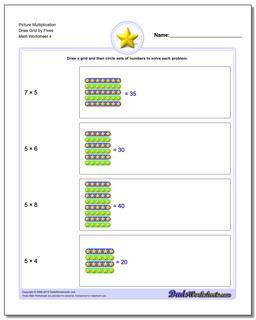# Math Worksheets: Picture Math Multiplication: Picture Math Multiplication: Picture Multiplication Draw Grid by Fives (Fourth Worksheet)## Picture Multiplication Draw Grid by Fives (Fourth Worksheet)

PropertyValue
DescriptionPicture Multiplication Draw Grid by Fives: Picture math multiplication worksheets where the student must draw the grid to solve the problem. (Fourth Worksheet)
Resource TypeWorksheet